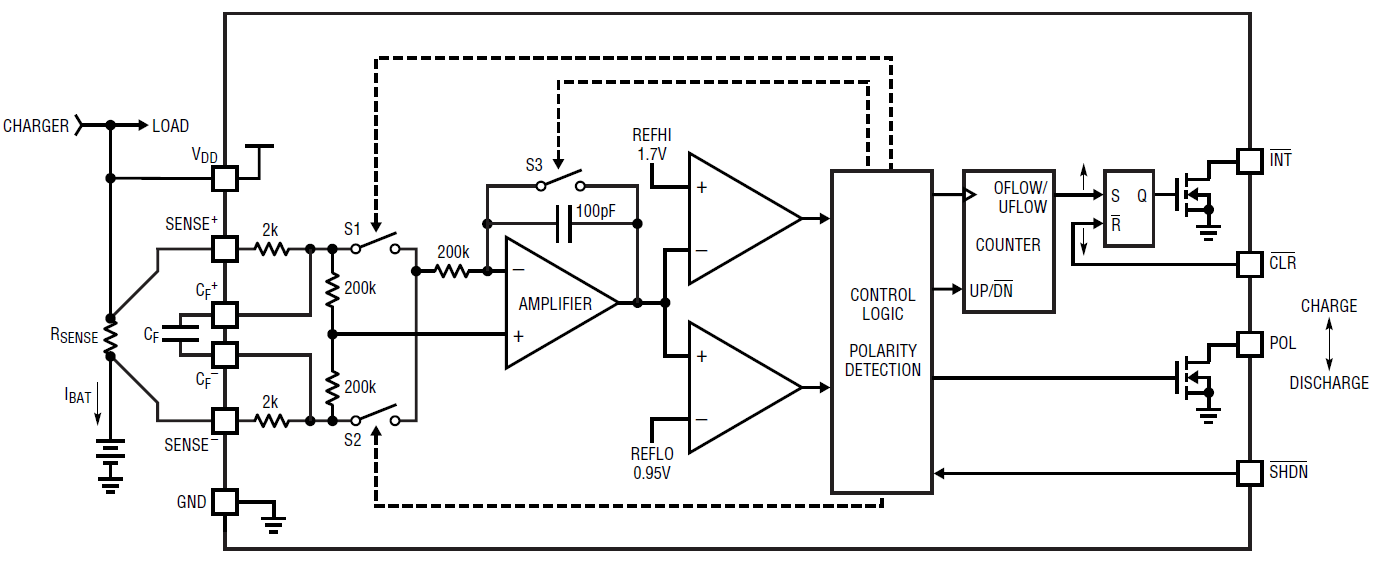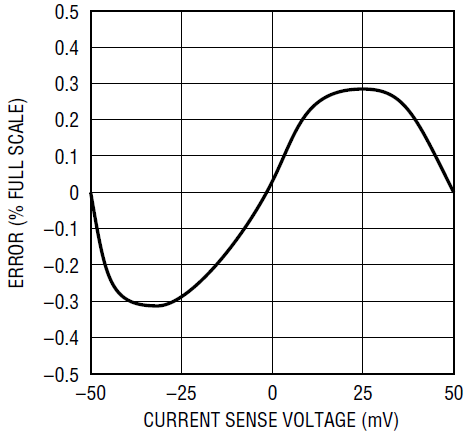# An Accurate Battery Gas Gauge

Introduction

A battery fuel gauge can be implemented in a variety of ways. The most popular is to derive the remaining battery capacity from the battery voltage. This method has advantages in that it is easy to implement and relatively low in cost, but it does have one major drawback: It is relatively inaccurate. Battery voltage has, at best, an inconsistent relationship to battery capacity—the relationship varies greatly depending on battery discharge rate and temperature.

The latest portable devices, though, require more accurate battery gas gauging. For instance, a portable computer or PDA may need to save data, or state information, and shut down when the battery reaches a critical discharge point. Accurate prediction of this point allows the device to safely run longer on battery power. For applications that require accurate gauging, the LTC4150 coulomb counter is a compact and easy-to-implement solution.

The LTC4150 measures the charge flowing into and out of the battery through a sense resistor. A voltage-to-frequency converter transforms the current sense voltage into a series of output pulses. Each pulse corresponds to a fixed quantity of charge flowing into or out of the battery. The device indicates the charge polarity as the battery is depleted or charged. The status of the battery can be accurately predicted by a microcontroller, connected via a simple 1-wire or 2-wire interface.

Precision Integrator Enables Charge Measurement

Charge is the time integral of current. The LTC4150 measures battery current by monitoring the voltage developed across a sense resistor and then integrates this information to determine charge. The block diagram shown in Figure 1 shows how.Figure 1. LTC4150 block diagram shows how measured current, at the sense resistor, is integrated and converted to an integer count of charge.

The current measurement is filtered by capacitor CF connected across CF+ and CF– pins. This averages all fast changes in current arising from ripple, noise and spikes in the load, charge current, or Burst Mode® operation of a switching regulator. The filter’s output is applied to an integrator with the amplifier and 100pF capacitor at its core. Switches S1 and S2 reverse the ramp direction once the integrator’s output reaches the REFHI or REFLO levels. By observing the condition of S1, S2, and the ramp direction, the polarity is determined.

A counter is used to effectively increase the integration time by a factor of 1024, greatly reducing microcontroller overhead required to service the interrupts from the LTC4150. At each counter underflow or overflow, the INT output latches low, while simultaneously, the POL output is latched to indicate the polarity of the charge count. Once the interrupt is recognized, the microcontroller resets INT output with a low going pulse at CLR pin. To simplify the connections, INT and CLR pins can also be connected together. In this case, the interrupt signal lasts at least 1µs, enough time for the microcontroller to register the data, before the INT pin resets automatically.

Coulomb Counting

The LTC4150’s transfer function is quantified as a voltage to frequency gain GVF, where the output frequency is the number of interrupts per second and the input voltage is the voltage VSENSE across the SENSE+ and SENSE– pins. The number of interrupts per second is:

Where:

VSENSE = 50mV for the LTC4150, therefore:

Since I • t = Q, the coulombs of battery charge per INT pulse (interrupt interval) can be derived from Equation 4:

Battery capacity is most often expressed in ampere-hours:

Combining Equations 4 and 5:

or

The charge measurement can then be scaled with a microcontroller.

High Side Sensing up to 8.5V

Figure 2 shows a typical application design for a 2-cell lithium-ion battery system with 500mA of maximum load current. Using Equation 2 to calculate RSENSE = 50mV/0.5A = 0.1Ω. With RSENSE = 0.1Ω, Equation 6 shows that each interrupt corresponds to 0.085mAh of charge with GVF = 32.55 Hz/V. A battery with 850mAh of capacity takes a total of 10,000 INT assertions to fully charge or discharge.

The LTC4150 can be shut down, when not needed, to a low current mode (1.5µA max) reducing the drain on the battery.

Accurate Prediction of Battery Capacity

The factors that affect the accuracy of the capacity prediction are the input offset voltage, the integral nonlinearity error (INL), the tolerance of the sense resistor, and the self-discharge of the battery. The self-discharge rate of a Li-Ion type of battery is around 2%–4% per month at room temperature. The LTC4150 has 0.3% of INL error across the input and common mode range, see Figure 3, and 150µV of input offset voltage.Figure 3. Integral nonlinearity of the LTC4150 is within 0.3% over the entire sense voltage range.

Conclusion

The LTC4150 offers a simple and compact solution for high side coulomb counting/battery gas gauging for battery voltages up to 8.5V(2-cell Li-Ion or 6-cell NiCd or NiMH batteries). The only required external components are the sense resistor and a filter capacitor to average out transient events and ripple current.